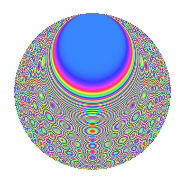# Properties

 Label 2100.2.lLevel 2100 Weight 2 Character orbit l Rep. character $$\chi_{2100}(1499,\cdot)$$ Character field $$\Q$$ Dimension 216 Sturm bound 960

# Related objects

## Defining parameters

 Level: $$N$$ $$=$$ $$2100 = 2^{2} \cdot 3 \cdot 5^{2} \cdot 7$$ Weight: $$k$$ $$=$$ $$2$$ Character orbit: $$[\chi]$$ $$=$$ 2100.l (of order $$2$$ and degree $$1$$) Character conductor: $$\operatorname{cond}(\chi)$$ $$=$$ $$60$$ Character field: $$\Q$$ Sturm bound: $$960$$

## Dimensions

The following table gives the dimensions of various subspaces of $$M_{2}(2100, [\chi])$$.

Total New Old
Modular forms 504 216 288
Cusp forms 456 216 240
Eisenstein series 48 0 48

## Trace form

 $$216q - 8q^{4} + O(q^{10})$$ $$216q - 8q^{4} + 8q^{16} + 60q^{24} + 64q^{34} + 12q^{36} + 48q^{46} + 216q^{49} - 84q^{54} + 96q^{61} - 80q^{64} + 92q^{66} + 48q^{69} + 48q^{76} + 16q^{81} + 72q^{94} - 44q^{96} + O(q^{100})$$

## Decomposition of $$S_{2}^{\mathrm{new}}(2100, [\chi])$$ into newform subspaces

The newforms in this space have not yet been added to the LMFDB.

## Decomposition of $$S_{2}^{\mathrm{old}}(2100, [\chi])$$ into lower level spaces

$$S_{2}^{\mathrm{old}}(2100, [\chi]) \cong$$ $$S_{2}^{\mathrm{new}}(60, [\chi])$$$$^{\oplus 4}$$$$\oplus$$$$S_{2}^{\mathrm{new}}(300, [\chi])$$$$^{\oplus 2}$$$$\oplus$$$$S_{2}^{\mathrm{new}}(420, [\chi])$$$$^{\oplus 2}$$

## Hecke characteristic polynomials

There are no characteristic polynomials of Hecke operators in the database